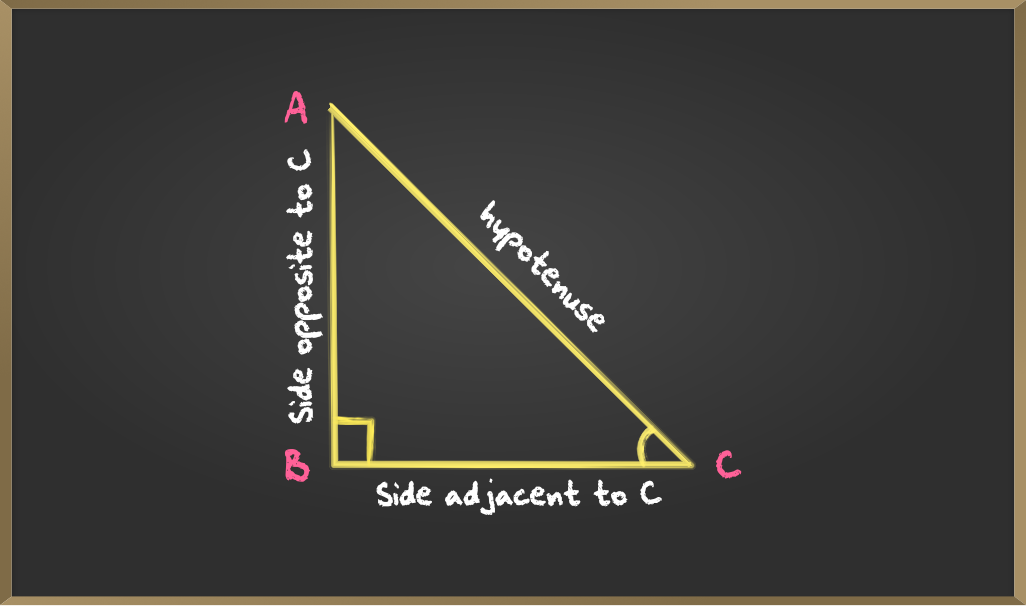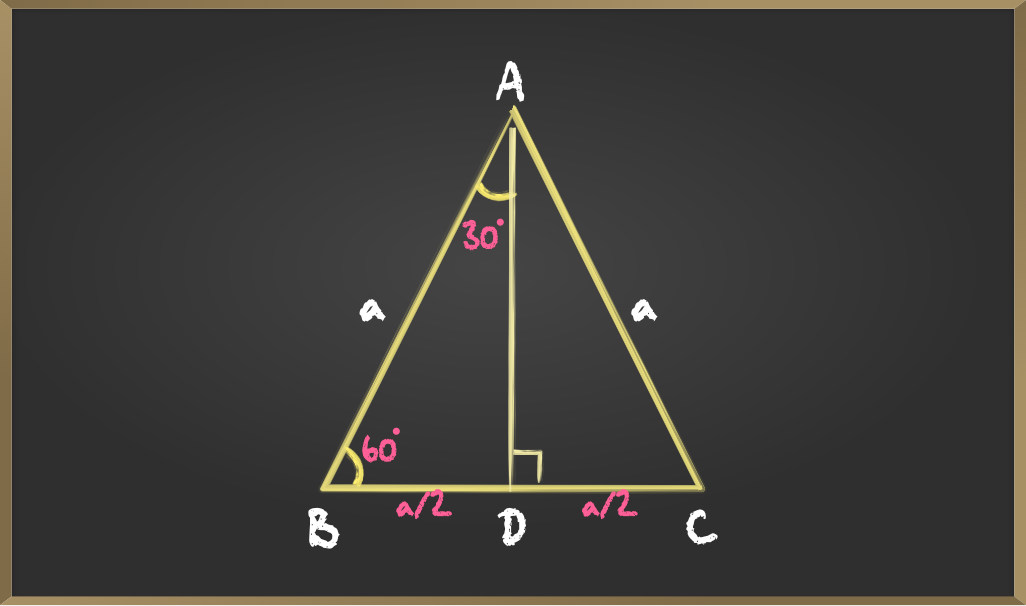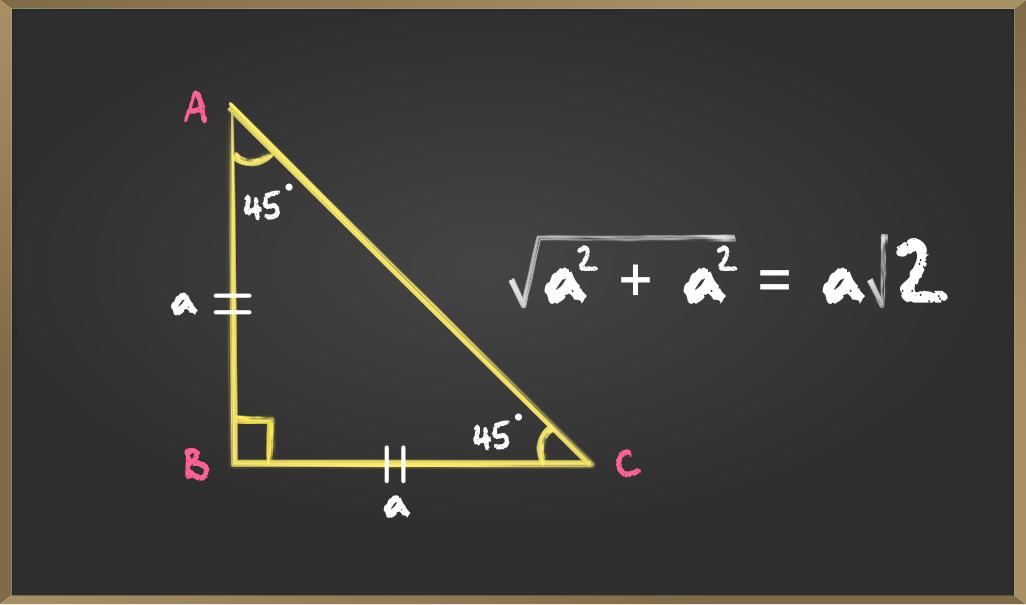# Trigonometric ratios of some Specific Angles

Trigonometry is all about triangles or to be more precise the relationship between the angles and sides of a triangle (right-angled triangle). In this article, we will be discussing the ratio of sides of a right-angled triangle concerning its acute angle called trigonometric ratios of the angle and find the trigonometric ratios of specific angles: 0°, 30°, 45°, 60°, and 90°.
Consider the following triangle,The side BA is opposite to the angle ∠BCA so we call BA the opposite side to ∠C and AC is the hypotenuse; the other side BC is the adjacent side to ∠C.

### Trigonometric Ratios of angle C

Sine: Sine of ∠C is the ratio of the side opposite to C (BA) to the hypotenuse (AC).Cosine: Cosine of ∠C is the ratio of the side adjacent to C (BC) and the hypotenuse (AC).Tangent: The tangent of ∠C is the ratio between the side opposite (BA) and adjacent to C (BC).Cosecant: Cosecant of ∠C is the reciprocal of sin C therefore it is the ratio of the hypotenuse (AC) to the side opposite to C (BA).Secant: Secant of ∠C is the reciprocal of cos C therefore it is the ratio of the hypotenuse (AC) to the side adjacent to C (BC).Cotangent: Cotangent of ∠C is the reciprocal of tan C that is the ratio of the side adjacent to C (BC) to the side opposite to C (BA).### Finding trigonometric ratios for angles 0°, 30°, 45°, 60°, 90°

Considering the length of the hypotenuse AC = a, BC = b and, BA = c.

A. For angles 0° and 90°

If angle A = 0°, the length of the opposite side would be zero and hypotenuse = adjacent side, and if A = 90°, the hypotenuse = opposite side. So, with the help of the above formulas for the trigonometric ratios we get –

if A = 0°if A = 90°Here some of the trigonometric ratios result as not defined as at the particular angle it is divided by 0 which is undefined.

B. For angles 30° and 60°

Consider an equilateral triangle ABC. Since each angle in an equilateral triangle is 60°, therefore,

∠A = ∠B = ∠C = 60°.∆ABD is a right triangle, right-angled at D with ∠BAD = 30° and ∠ABD = 60°,

Here ∆ADB and ∆ADC are similar as they are Corresponding parts of Congruent triangles (CPCT).Now we know the values of AB, BD, and AD, So the trigonometric ratios for angle 30° are,For angle 60°C. For angle 45°

In a right-angled triangle if one angle is 45° then the other angle is also 45° thus, making it an isosceles right-angle triangle.If the length of side BC = a then length of AB = a and length of AC(hypotenuse) is a√2 using Pythagoras Theorem, then### All values

Whether you're preparing for your first job interview or aiming to upskill in this ever-evolving tech landscape, GeeksforGeeks Courses are your key to success. We provide top-quality content at affordable prices, all geared towards accelerating your growth in a time-bound manner. Join the millions we've already empowered, and we're here to do the same for you. Don't miss out - check it out now!

Related Tutorials